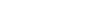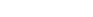Unit Conversion Calculator in JavaScript

## www.PMEL.org      Physical Conversions

from 1 to 15 digits

From

To

Conversion Factor

Value

Units Value Units Multiply by

English units of mass, weight and force are Avoirdupois; metric units are International metric.
Click on From Value blank, type Value and select From and To Units.

## www.PMEL.org     Temperature Conversions // <!-- function Cvalue(num,index1,index2){ var temp=0; if(index1==1){ if(index2==1)temp=num; if(index2==2)temp=9/5*num+32; if(index2==3)temp=1*num+273.15; if(index2==4)temp=9/5*num+32+459.67; } if(index1==2){ if(index2==1)temp=5/9*(num-32); if(index2==2)temp=num; if(index2==3)temp=5/9*(num-32)+273.15; if(index2==4)temp=1*num+459.67; } if(index1==3){ if(index2==1)temp=num-273.15; if(index2==2)temp=9/5*(num-273.15)+32; if(index2==3)temp=num; if(index2==4)temp=9/5*(num-273.15)+32+459.67; } if(index1==4){ if(index2==1)temp=5/9*(num-32-459.67); if(index2==2)temp=num-459.67; if(index2==3)temp=5/9*((num-32)-459.67)+273.15; if(index2==4)temp=num; } return Math.round(temp*10000)/10000 } // -->

=

Click on blank and type in a value, then click outside the box.

# PMEL.ORG PHYSICAL CONVERSIONS

(Works best with Internet Explorer)“Illegitimi Non Carborundum”
(Works best with Internet Explorer)

# PMEL.ORG PHYSICAL CONVERSIONS

Unit Conversion Calculator in JavaScript

## www.PMEL.org      Physical Conversions

from 1 to 15 digits

From

To

Conversion Factor

Value

Units Value Units Multiply by

English units of mass, weight and force are Avoirdupois; metric units are International metric.
Click on From Value blank, type Value and select From and To Units.

## www.PMEL.org     Temperature Conversions // <!-- function Cvalue(num,index1,index2){ var temp=0; if(index1==1){ if(index2==1)temp=num; if(index2==2)temp=9/5*num+32; if(index2==3)temp=1*num+273.15; if(index2==4)temp=9/5*num+32+459.67; } if(index1==2){ if(index2==1)temp=5/9*(num-32); if(index2==2)temp=num; if(index2==3)temp=5/9*(num-32)+273.15; if(index2==4)temp=1*num+459.67; } if(index1==3){ if(index2==1)temp=num-273.15; if(index2==2)temp=9/5*(num-273.15)+32; if(index2==3)temp=num; if(index2==4)temp=9/5*(num-273.15)+32+459.67; } if(index1==4){ if(index2==1)temp=5/9*(num-32-459.67); if(index2==2)temp=num-459.67; if(index2==3)temp=5/9*((num-32)-459.67)+273.15; if(index2==4)temp=num; } return Math.round(temp*10000)/10000 } // -->

=

Click on blank and type in a value, then click outside the box.